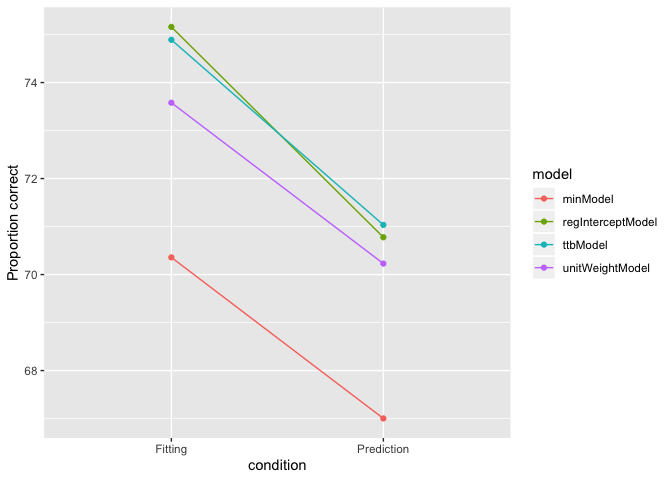# Reproducing Results

#### 2019-08-19

library(heuristica)

# Prior results

Heuristica can be used to reproduce results from prior research, making sure to have the same data set and heuristics. Consider the city population results on page 103 of Simple Heuristics That Make Us Smart (citation below). It reported the following percent correct for models fitting city populations– that is, when the models are given all 83 cities to fit and then are asked to estimate the poulation of the same 83 cities:

Regression Dawes Take The Best Minimalist
74 74 74 70

# Getting the same data and models

## Data set

To use the exact same data set as that research, we need city_population_original, even though it had some transcription errors from the almanac. (city_population corrected the transciption errors.)

## Models

Here is how the models from prior research map the heuristica models:

Regression Dawes’ model Take The Best Minimalist
regInterceptModel unitWeightModel ttbModel minModel

Two of the mappings are non-obvious.

regInterceptModel By default, regression models include the intercept, so prior research included it, and that maps to regInterceptModel. However, when comparing two estimates, the intercepts cancel out, so fitting the intercept just wastes a degree of freedom. Future research should use regModel.

unitWeightModel Because the term “dawes model” did not catch on, this package uses the more commonly-known descriptive name, unitWeightModel. The models are the same.

(Likewise Franklin’s model from the book maps to validityWeightModel in heuristica.)

# Simulation

Now fit the models to the data. Regression will determine its beta weights, ttb will determine cue order, and unit weight linear and minimalist will determine cue direction (whether having a soccer team is associate with higher or lower population).

data_set <- city_population_original
criterion_col <- 3    # Population
cols_to_fit <- 4:ncol(data_set) # The 9 cues

reg <- regInterceptModel(data_set, criterion_col, cols_to_fit)
ttb <- ttbModel(data_set, criterion_col, cols_to_fit)
unit <- unitWeightModel(data_set, criterion_col, cols_to_fit)
min <- minModel(data_set, criterion_col, cols_to_fit)

To determin the percent of correct inference in fitting, pass the exact same data set into the percent correct function with the fitted models.

results <- percentCorrectList(data_set, list(reg, ttb, unit, min))
# Round values to make comparison easier.
round(results)
##   regInterceptModel ttbModel unitWeightModel minModel
## 1                74       74              74       70

The results match the results reported in the book– 74% correct for all models except minimalist, which got only 70% correct.

# Prediction

The code above simulates fitting. To simulate prediction, fit the models with a subset of the cities and run percentCorrect with the other cities. Then repeat for various ways to split the data and run summary statistics on the results. Below is a function to do this from the cross-validation vignette.

# Cross-validate the data over the vector of models, suing training-proportion
# of the data to train and the rest as a test set.  Outputs the mean percent
# correct for each model in fitting and in prediction.
crossV <- function(vec_of_models, criterion_col, cols_to_fit, data, reps, training_proportion){
fitting <- vector()
prediction <- vector()
for(i in 1:reps){

#randomly sample training and test row indexes
train <- sample(1:nrow(data), nrow(data)*training_proportion)
test <- setdiff(1:nrow(data), train)

#create training and test set
training_set <- data[train,]
test_set <- data[test,]

# If a regression is overdetermined (e.g. has too many columns(), it will
# drop the right-most columns.  To instead make it drop random columns,
# we shuffle the column order.
shuffled_cols_to_fit <- sample(cols_to_fit)

models<-list()
y <- 0
for (mod in vec_of_models) { #fit the models to the training_set
y <- y+1
models[[y]] <- mod(training_set, criterion_col, shuffled_cols_to_fit)
}

#calculate percentage of correct predictions
fittingAccuracy <- percentCorrectList(training_set, models)
predictionAccuracy <- percentCorrectList(test_set, models)
fitting <- rbind(fitting,fittingAccuracy)
prediction <- rbind(prediction,predictionAccuracy)
}

results <- (rbind(colMeans(fitting),colMeans(prediction)))
rownames(results) <- c("Fitting","Prediction")
results
}

Now use the function with the models and data defined above.

set.seed(0) # Use the same seed if you want to reproduce same results.
reps <- 200 # The book used 10,000 but that takes minutes.
training_proportion <- 0.5

results <- crossV(c(regInterceptModel, ttbModel, unitWeightModel, minModel), criterion_col, cols_to_fit, data_set, reps,training_proportion)
# Round values to make comparison easier.
round(results, 1)
##            regInterceptModel ttbModel unitWeightModel minModel
## Fitting                 75.2     74.9            73.6     70.4
## Prediction              70.8     71.0            70.2     67.0

Notice that

• Accuracy is always lower for prediction compared to fitting. (Predicting out of sample is harder than fitting a known sample.)
• ttbModel is more accurate than regression in prediction on the cities data set.

This result differs from some others for several reasons

• The book used a regression model with intercept, so that is what was used here. However, the example on the cross-validation page uses a non-intercept regression, so slightly different answers are expected.
• A few hundred reps are not enough for stable results. The book used 10,000.

The book does not actually report the prediction results of the cities data set individually, but the results are similar to the average results. Some of the individual plots in book also show the large accuracy drop for regression between fitting and prediction, since this data represents the point at 9.21 objects per cue (83 cities / 9 cues).

Below is a fitting vs. prediction plot but using just the cities data. The book shows this type of plot across all 20 data sets.

library(ggplot2)
library(reshape)
p <- melt(results)
colnames(p) <- c("condition","model","value")
ggplot(p, aes(x=condition, y=value, colour=model,group=model)) + geom_point() + geom_line() + ylab("Proportion correct")